Categories

## Drawing Nice Orbits

So, I have been working on Kepler Project again, and I thought I would write about some of the interesting challenges I have encountered and how I overcame them.

## The Shapes of an Orbit

Today, we will talk about drawing orbits. I will talk about the orbital computations themselves another time.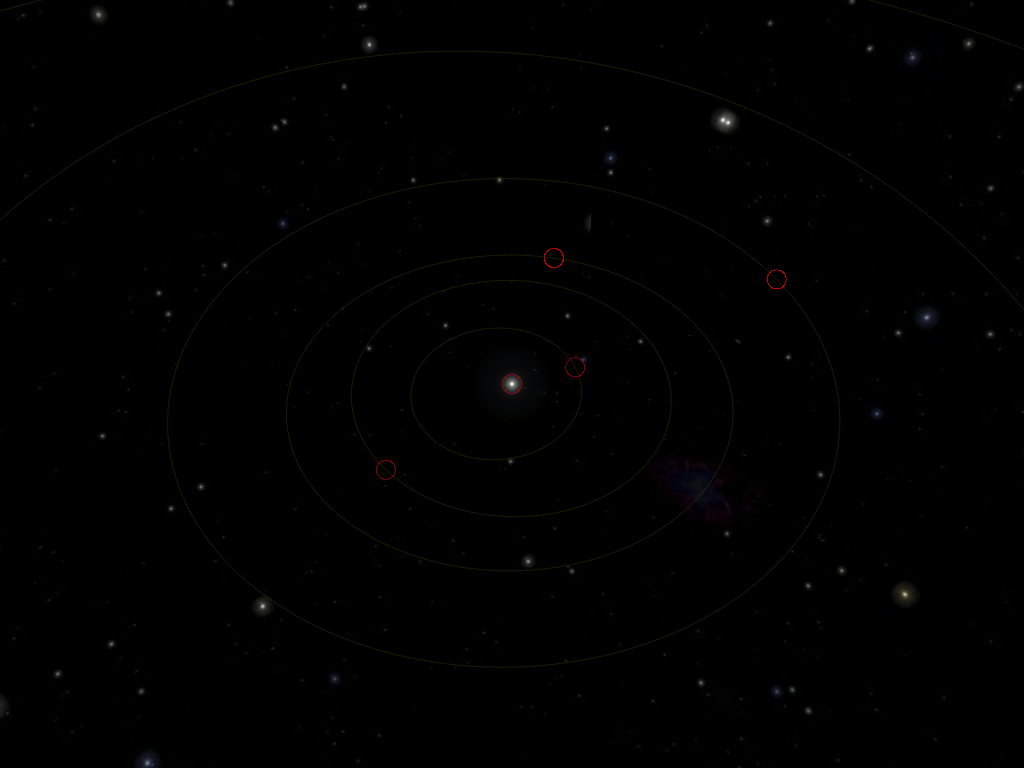Here, we can see the full orbits of the four terrestrial (i.e. rocky) planets: from interior to exterior, Mercury, Venus, Earth and Mars. In the middle, of course, is the Sun, and the outermost orbit partially visible here is that of Ceres, a dwarf planet whose orbit lies between those of Mars and Jupiter. The red circle show the current (as of 2021-01-27) location of the planet in their orbits.

The planets of the Solar System follow an ellipse as they revolve around the Sun. They are fairly close to a circle, except for the notable exception that is Mercury: in the screenshot above, you can tell that the Sun is not in the middle of Mercury’s orbit (the innermost one). In fact, the trajectory of Mercury draws an ellipse whose focus is the Sun.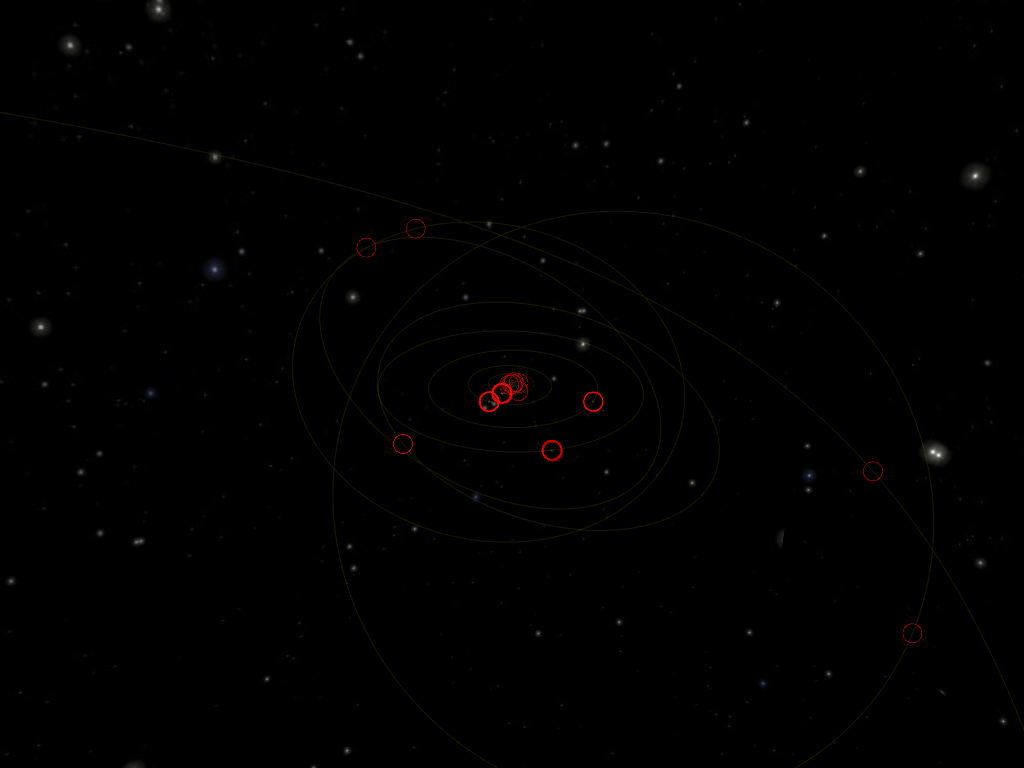Zooming out a bit, we see that there are quite a few dwarf planets orbiting the Sun from far away a bit haphazardously. The four regular orbits in the middle are those of the gas giants: from interior to exterior, Jupiter (whose Orbit is partially hidden behind all the red circles of the inner planets), Saturn, Uranus and Neptune. We find the dwarf planet Eris the bottom-right corner, and Sedna, a bit above, whose orbit is so extended, it takes ten thousand years (Earth revolutions) to complete a full revolution in its orbit.

From the first image, and from the usual depictions of the Solar System, you could think that the orbits are always neatly arranged. But that this becomes increasingly inaccurate as we pull farther away from the Sun.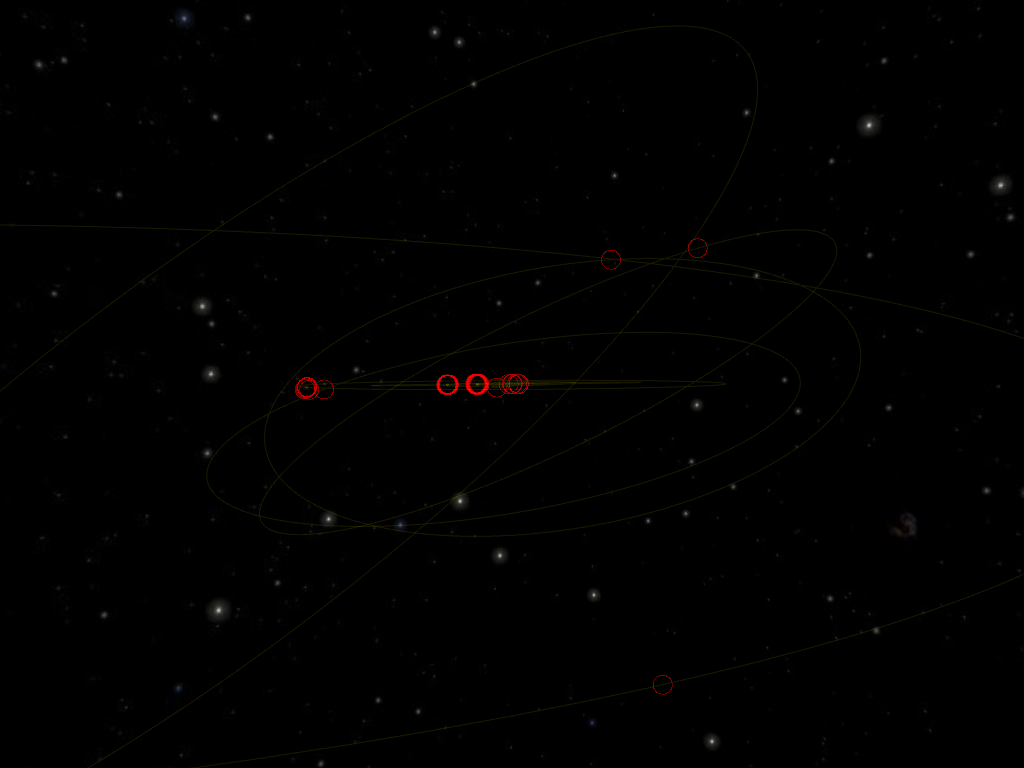In this side-view, we can better appreciate that the orbits of the eight planets and Ceres lie almost in the same plane, while the orbits of the outer dwarf planets have a much more pronounced inclination.

From this, we know we are going to need to draw ellipses shapes (eccentricity) and sizes (I use the periapsis to characterize the size of the orbits) in all kinds of orientations (since there are three spatial dimensions, we will need three parameters; I use longitude of the ascending node, inclination and argument of periapsis).

## Drawing an Ellipse

GPUs let you draw points, line segments and triangles. And that’s pretty much it. The point is that these primitives let you draw anything if you use enough of them. By restricting ourselves to the simplest primitives, we let the GPU designers focus on doing one thing well. Of course, there is a huge swath of features to help you draw millions of them efficiently, but everything you see in a 3D game is pretty much drawn with textured triangles.

So, how do we draw an ellipse when we can only draw line segments? Well, you pick points on the ellipse and connect them together to make a polygon that approximates the real curve: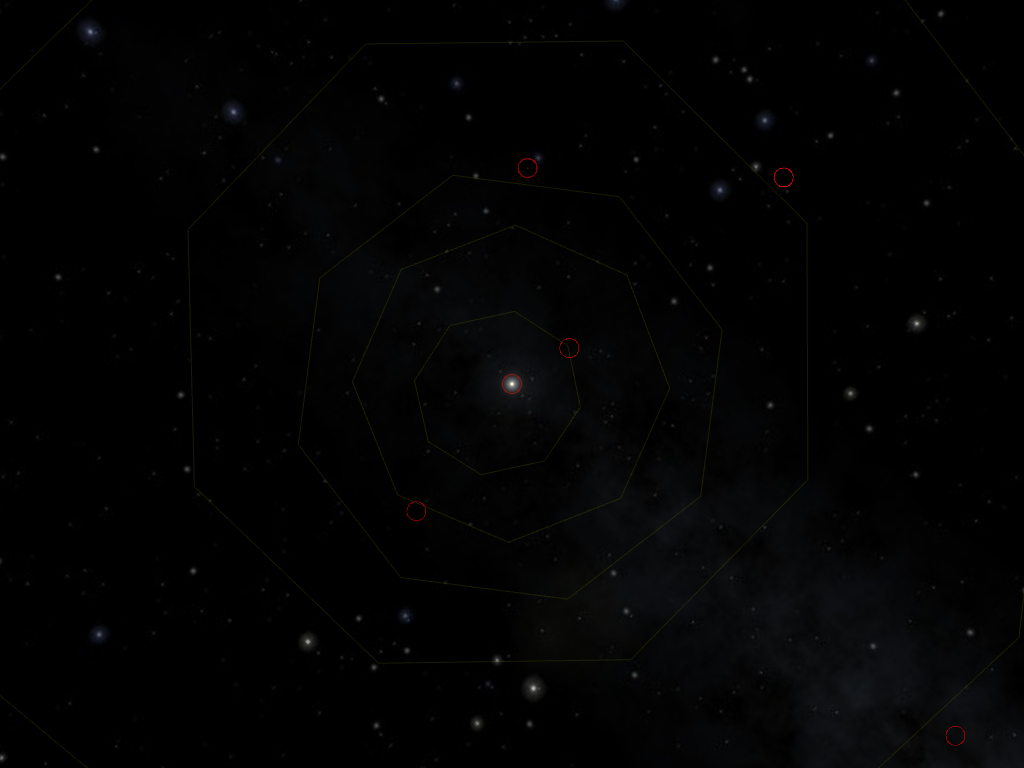Here, with only 8 segments to draw an orbit, you might just tell something is off. By the way, notice that, as a consequence, the objects (in the red circles) are not on the orbit we’ve drawn; it will come up later.

If we use enough segments, the illusion becomes good enough: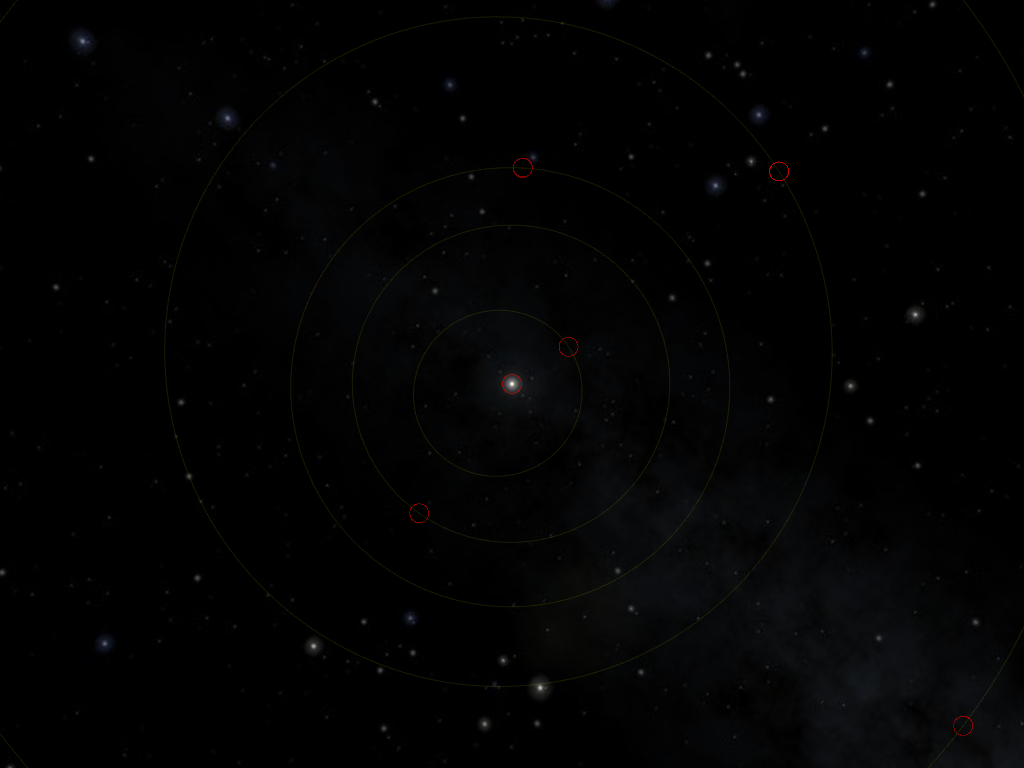In Kepler Project, each orbit is drawn using 256 segments. It could become visible at a high enough resolution, but it does the job.

So, we’re done, right? Not quite. We have only looked as near-circular orbits. Look at what happens with a more eccentric (more elliptical) orbit: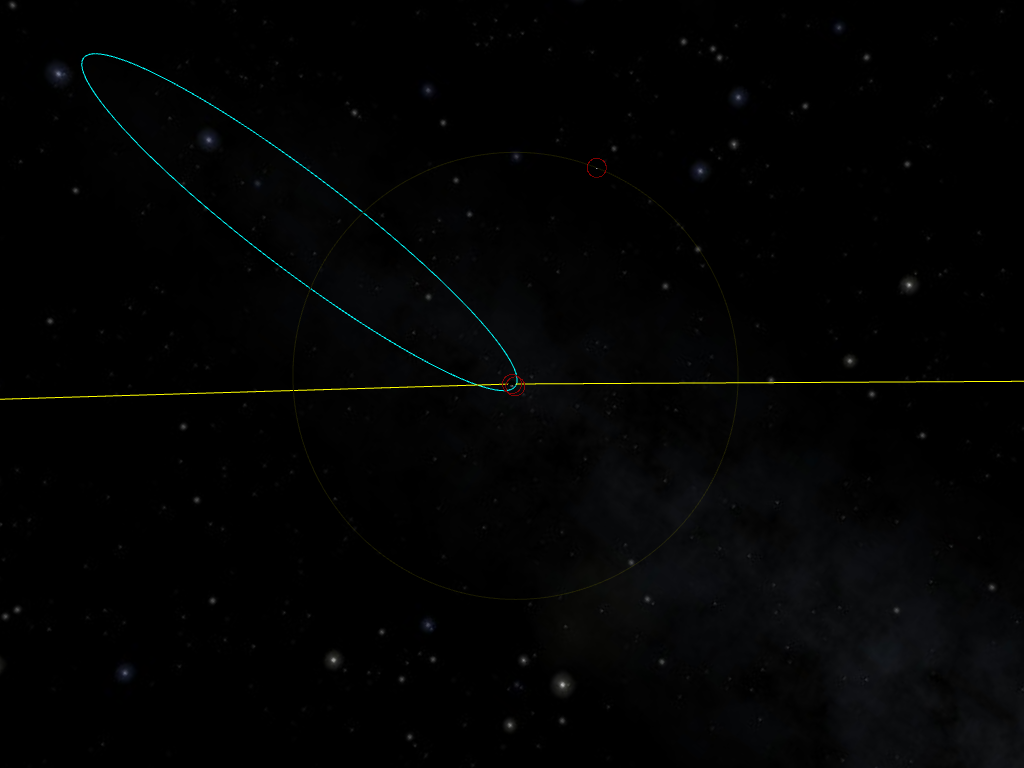I have set the apogee to 924 Mm, at the edge of Earth’s sphere of influence. The orbit has an eccentricity of 0.985.

## Drawing a Nice Ellipse

So far so good. But let’s zoom-in closer to Earth and the perigee of our orbit: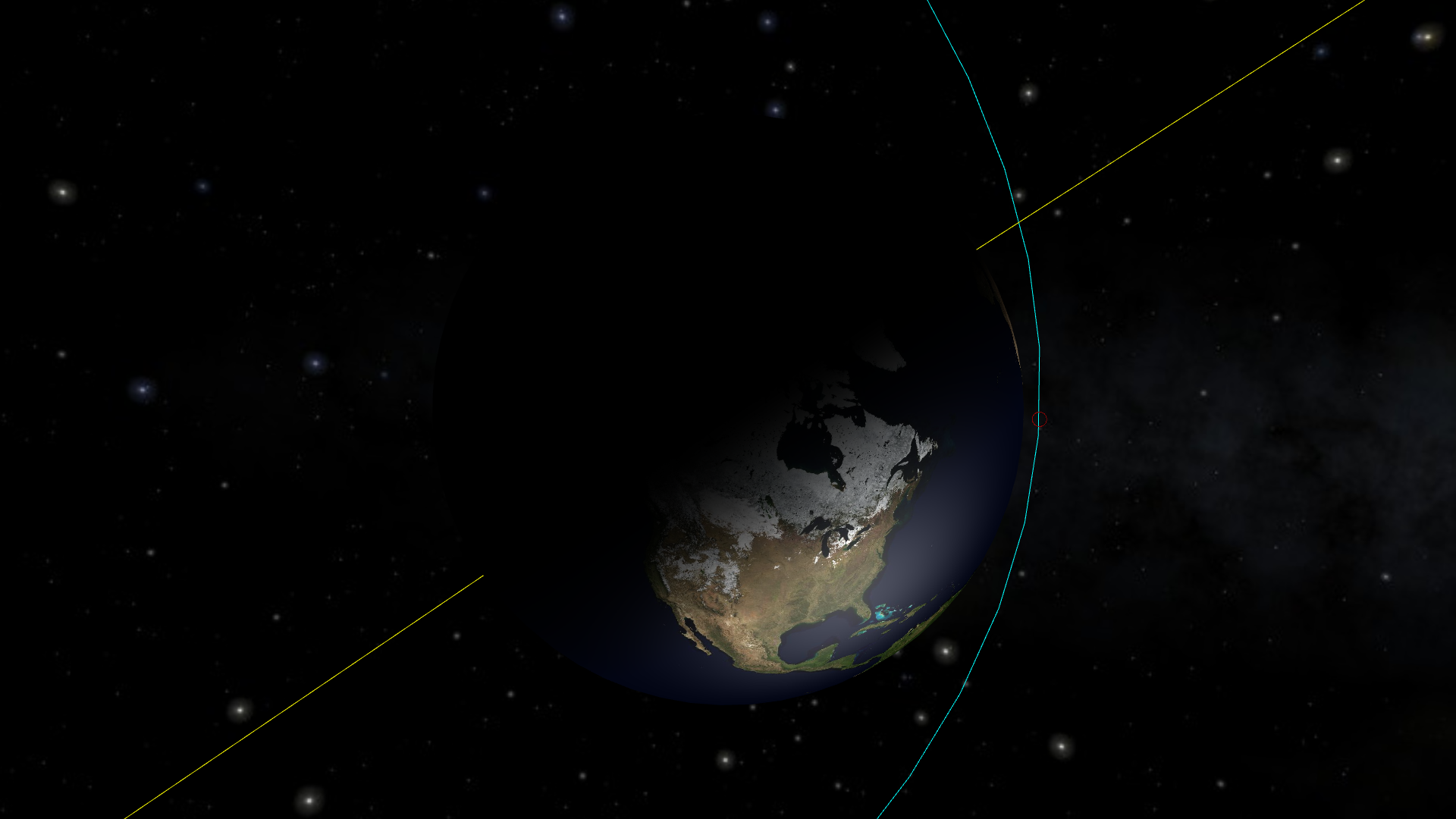The line segments are clearly visible on the right. It would only get worse with more eccentric orbits.

This is not good enough for me. I might accept glitches are extreme and unrealistic orbits, but this is not the case here. We could of course raise the number of segments used to draw the ellipse, and it would work (here, 1024 seems enough). But we should have ourselves why. Why do we need to use more segments in this case? Let’s have a look: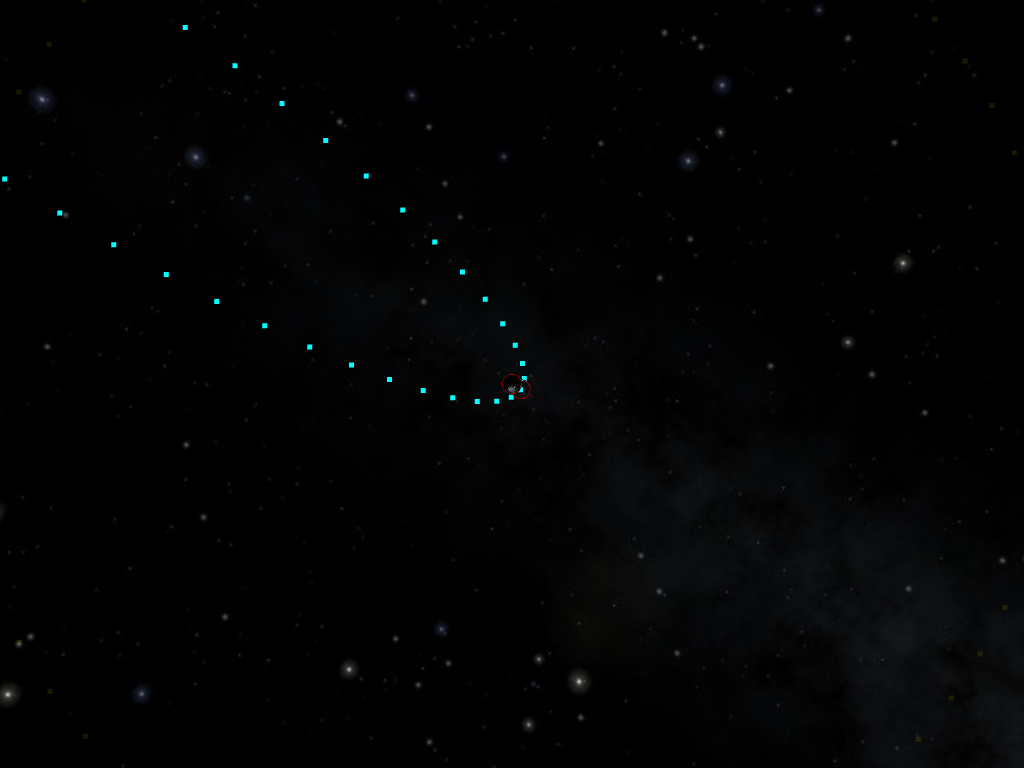I switched to only drawing the points (well, big points), and only 64 of them per orbit.

The curve is pretty tame in the middle of the ellipse (on the left of the image), but much stronger closer to the extremities (in the center of the image). From this, we should notice that we have more than enough points in the middle, and not enough at the extremities. If we distributed them better, we would not need more points!

How do we do that?

If found a suggestion on Stack Overflow. But the reasoning is not very clear to follow, so I expose it here. We want to make sure the angle between two consecutive line segments is small enough so that it is not visible.

First trick: We can simplify things by reframing the problem as making sure the angle between two consecutive segments is always the same. To understand why it makes sense, imagine all the angle between consecutive segments is always the same; then, if you decrease the angle between two consecutive segments, you have to increase at least one angle between two other consecutive segments (for a fixed number of segments of course).

Second trick: to avoid the annoying part of finding a formula for the angle between two segments depending on the placement of their extremities, we will actually look at the tangents to the ellipse in the points we choose.

Third trick: we already know we want a constant angle difference between two consecutive tangents, but that means we already pretty much know this angle: 2 π / n where n is the number of segments (note: strictly, we should not assume one of the points will have an angle of 0; but we’re going to want to have a point at the periapsis anyway).

These tricks allow us to forget entirely about the line segments and just look at the tangent of the orbit in a given point: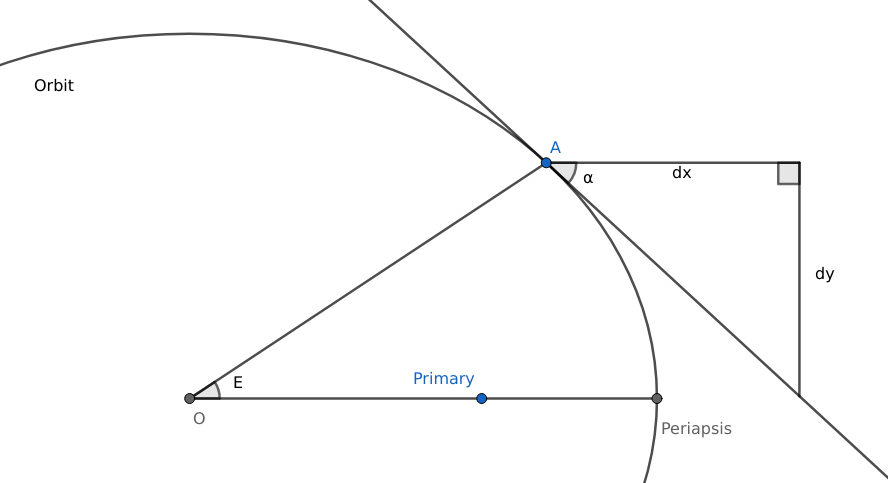The primary is one of the foci of the ellipse. The center of the ellipse, though, does not map to anything in particular and could very well be in outer space. E is short of “eccentric anomaly”, which is the angle between the periapsis and the object measured at the center (hence “eccentric”). Another common angle is the “true anomaly”, which is the angle between the periapsis and the object, measure at the primary, usually the actual point of reference (hence “true”).

In the figure above, we know α, but we need to know E. Once we know E, the actual coordinates of A can be calculated using the parametric formula for the ellipse: x = a cos E and y = b sin E. And now we use:

Just kidding, we use applied mathematics witchcraft:

latex \begin{aligned}\tan \alpha &= \frac {\mathop{dy}} {\mathop{dx}} = \frac { \frac {\mathop{dy}} {\mathop{dE}} } { \frac {\mathop{dx}} {\mathop{dE}} } = \frac { \frac {\mathop{d}} {\mathop{dE}} b \sin E } { \frac {\mathop{d}} {\mathop{dE}} a \cos E } \\ &= \frac { b \cos E } { – a \sin E } = – \frac b { a \tan E } \end{aligned}&s=4

Now, solving for E, we get:

$latex E = – \arctan \frac b { a \tan \alpha }&s=4$

This formula is not convenient because of the discontinuity around α = 0, so we use some more trigonometric sorcery to get to:

$latex E = \frac \pi 2 – \arctan \frac a b \tan \alpha&s=4$

Let’s look at the effect of using this formula on the repartition of our points: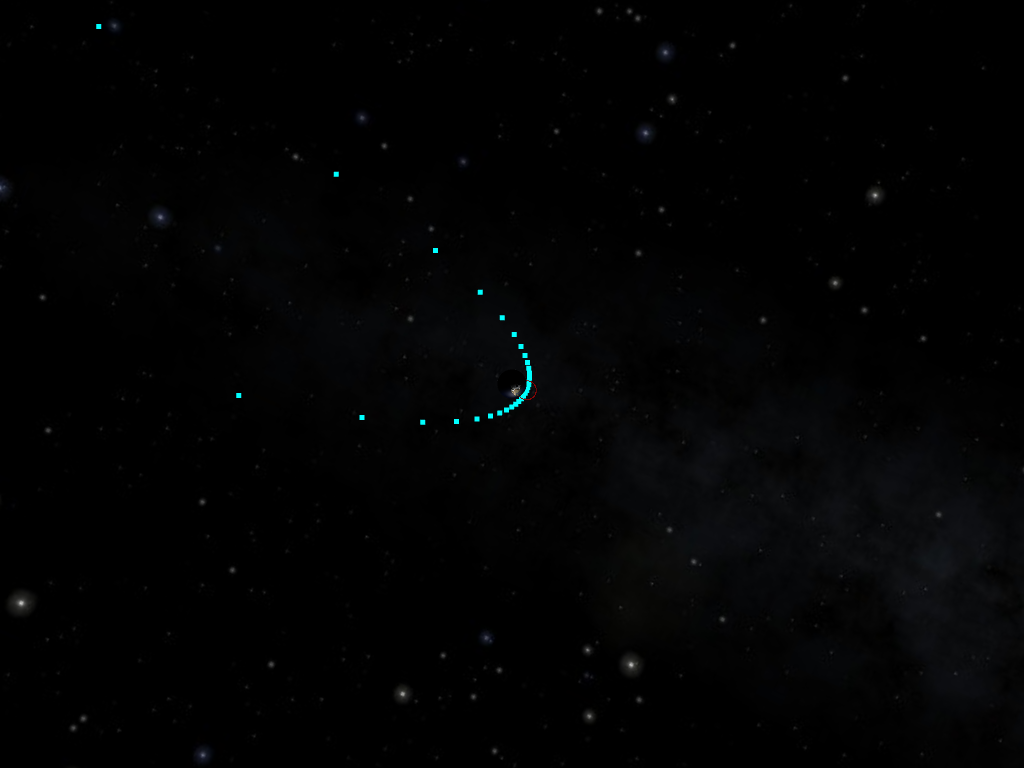Still with 64 points. They are much more concentrated around the perigee (and apogee on the other side, not visible here)

And the final result when switching back to line segments: